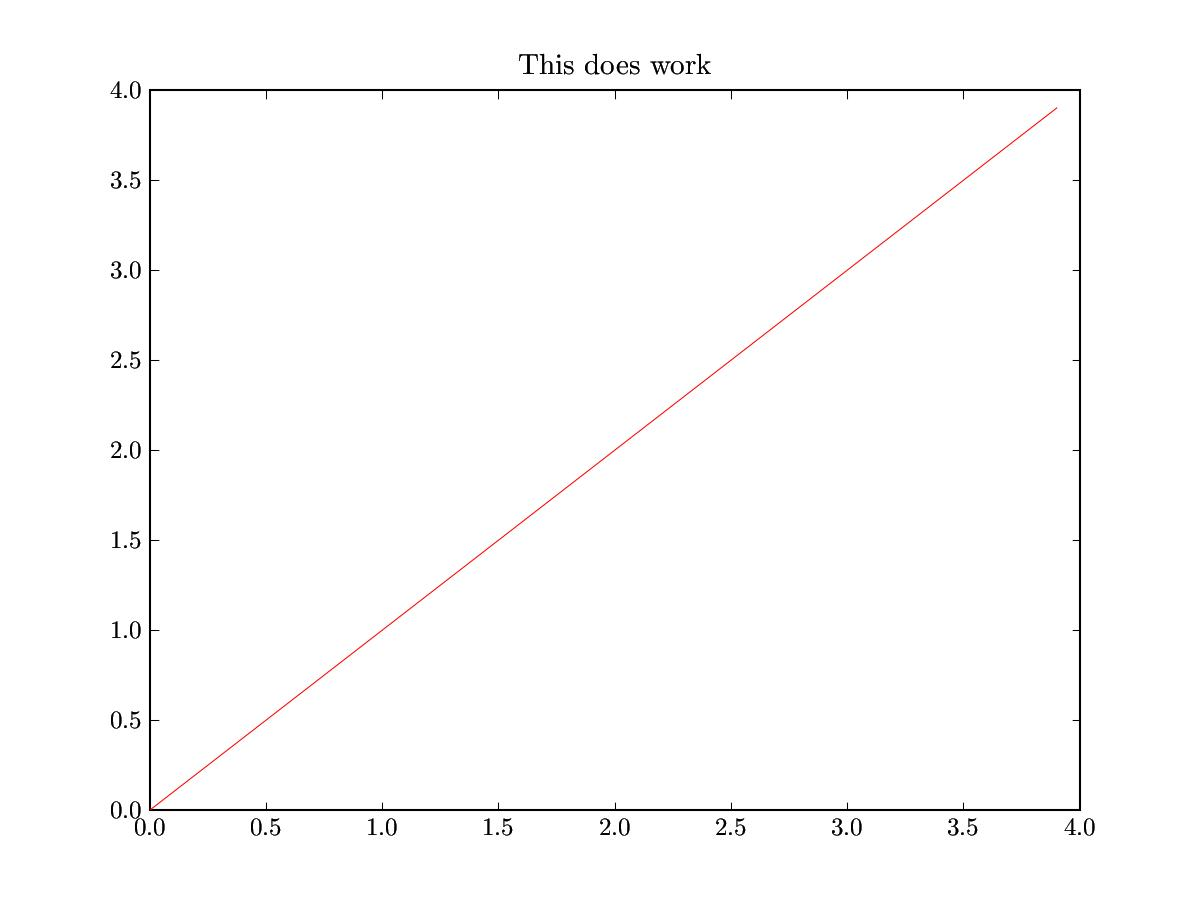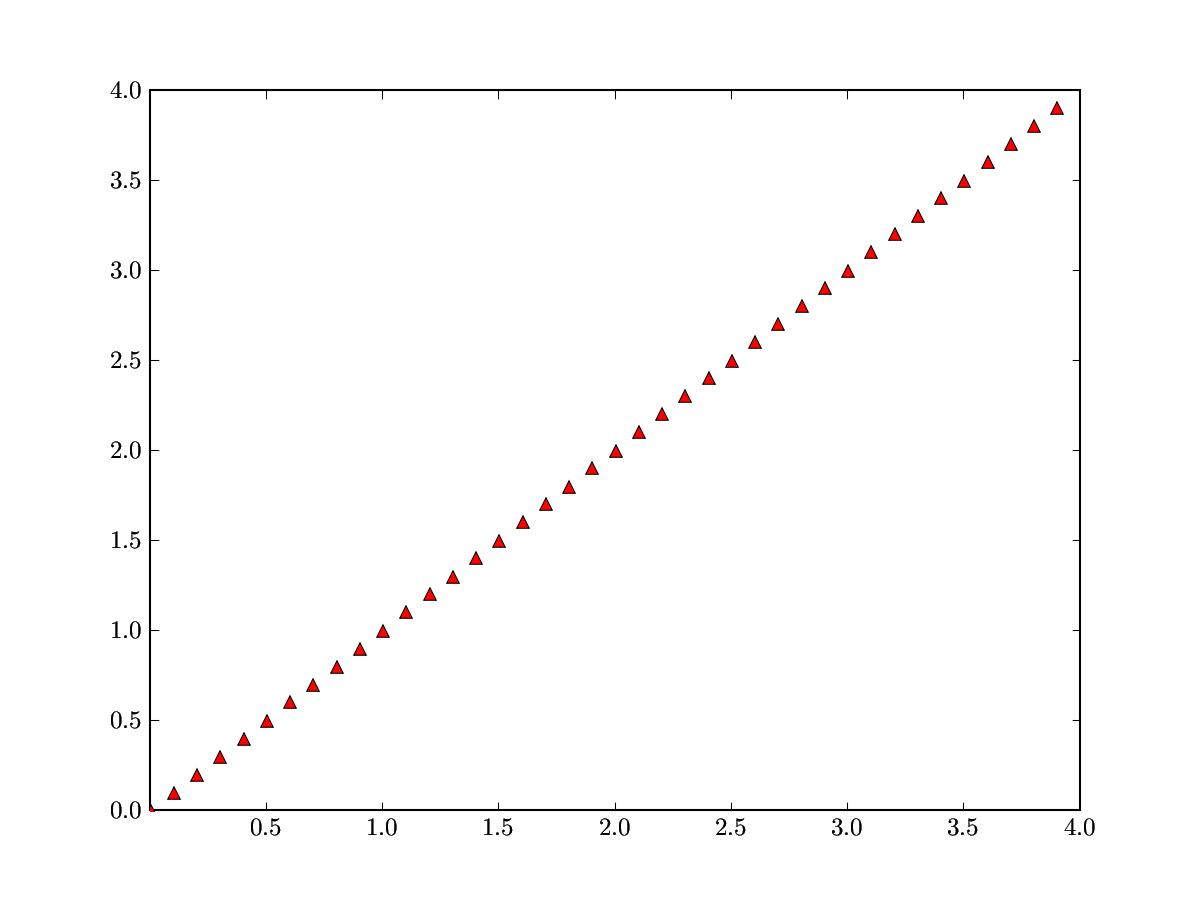# possible bug -- matplotlib 0.84

Here are two almost identical bits of code. The first one prints the title. The second doesn’t. It’s really baffling.···

from pylab import *
import string
from matplotlib import rc

rc(‘text’, usetex=True)

## x = arange(0,4,0.1) y = x figure() plot(x,y,‘r’) title(r’This does work’) show()

Now, all I do is change the plot style to print triangles instead of
just a line. (Note the carat in the style string) The title no longer
prints!

from pylab import *
import string
from matplotlib import rc

rc(‘text’, usetex=True)

## x = arange(0,4,0.1) y = x figure() plot(x,y,‘r^’) title(r’This does NOT work’) show()

In both cases, I get the following error message:

end from FAM server connection
failed to read() from server connection

Other experiments also lead to the same conclusion: The title only
prints when the plot style is only lines. Incidentally, both these
pieces of code work fine on matplotlib 0.82. Ideas?

Regards,
Dev